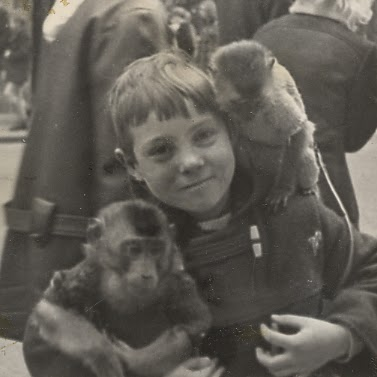# Magnification of a real image formed by a concave lens

2,103

I note that you are using the Cartesian Convention, in which distances are +ve measured to the right of the lens. Light is travelling left to right.

I agree with region 1 (real object, virtual image, diminished). However, in region 2 I get virtual object and real image ($v>0$), magnified. As you point out, this should be the opposite of region 1.

Region 3 is virtual object ($u>0$), virtual image ($v<0$). Magnification can be $<1$ ($u>f/2$), $=1$ ($u=v=f/2$) or $>1$ ($u<f/2$). This follows quite easily from your graph.

1. In region 3 the image can be magnified or diminished, depending on the object position.

2. The image in region 3 is virtual ($v<0$). Your calculation with Newton's formula is correct. It is your interpretation of your graph which is at fault.

Share:
2,103Author by

### Alu

Updated on December 16, 2022

•Alu 5 months

Graph of 1/u and 1/v for Concave Lens:

Lens Formula: $$\frac{1}{v}-\frac{1}{u}=\frac{1}{f}$$ Since, f is negative for a concave lens (diverging lens), re-writting the equation $$\frac{1}{v}=\frac{1}{u}-\frac{1}{f}$$Now, we know from the graph that for a real object it's image will be virtual(formed behind the lens between f and optical center) and diminished too since $\frac{1}{v}$ is greater than $\frac{1}{u}$ i.e v is smaller than u and $$m=\frac{v}{u}$$ Represented by the region 1.

Also, from the principle of reversibility of light, a virtual object being formed between focus and optical center will have its image formed behind the lens i.e virtual and magnified. Represented by the region 2.

And now what about a virtual object being formed beyond focus (region 3)? From the graph, I think it will be real but magnified for some region and diminished for some.So as to get some clarity I used Newtonian Thin Lens Equation $$x_1\cdot x_2=f^2$$ where

$x_1$ is the distance of object from the focus

$x_2$ is the distance of the image from the focus

Now since $x_1$ is greater than 2f $x_2$ should be less than f/2 which will result in the formation of a virtual image and not a real image, which is conflicting from the result we obtained from the graph.

To summarize, my question is as follows:

1. Whether the image of the virtual object will be magnified or diminished or both?
2. The image formed will be real for sure but from Newtonian Thin Lens Equation I am getting a virtual image. So what am I doing wrong?
•-1. Have you searched the internet? eg using your title I get this as #2 hit : physicsclassroom.com/class/refrn/Lesson-5/…
•It doesn't talk about the image formation of a VIRTUAL object.
•youtube.com/watch?v=9kyD3aiO70c. This tutorial uses a convex lens, but the principles of ray-tracing for a virtual object are the same.
•Do you have a particular situation in mind? If so, please can you post the details and show your attempt. It is easiest to explain using the problem you are struggling with, and the step you are confused about.
•Yes I am aware you are asking about a concave lens. That is why I said that the principles are the same as those discussed in the youtube video.
•@sammygerbil I have posted my detailed question.
•You have posted your solution, but not the question.
•It's their at the last.
•Oh sorry, your problem is trying to interpret the graph.
•In Cartesian Convention distances are measured +ve in the direction of incident light and not in the right.Also, I have pointed out the principle of reversibility of light from which this case will be similar to region 1.Can you explain the region 3 with the help of Newtonian Thin Lens Equation?
•Yes, I am assuming light is travelling left to right, which is usual. Your calculation using Newton's formula appears to give the correct answer.
•••••